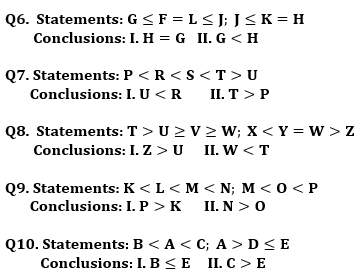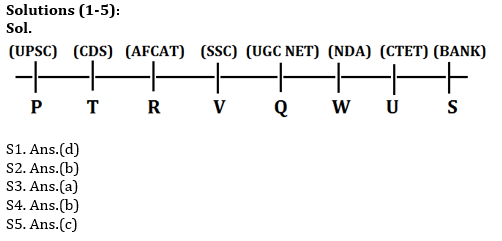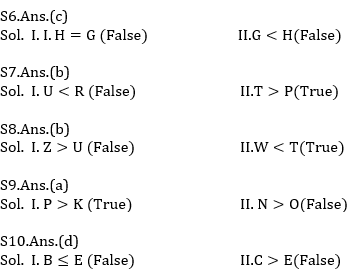Latest Banking jobs   »   Revision Test Reasoning Quiz for All...

# Revision Test Reasoning Quiz for All Banking Exams- 7th June

Directions (1-5): Study the following information carefully and answer the question given below.
Eight persons are sitting in a straight line and all are facing north direction. Each of them prepares for different competitive exam. S prepares for Bank and sits third to the right of Q. R sits immediate left of V who prepares for SSC. The one who prepares for AFCAT sits second to the left of Q. The one who prepares for UPSC sits third to the left of V. W prepares for NDA and he is an immediate neighbour of U who does not prepare for AFCAT. V is not an immediate neighbour of S. Persons who prepare for CDS and UPSC sit together. T sits third to the left of the one who prepares for UGC NET. W is not an immediate neighbour of S. P does not prepare for CTET.

Q1. Who among the following person prepare for CTET exam?
(a) T
(b) R
(c) W
(d) U
(e) None of these

Q2. Who among the following person sits third to the right V?
(a) the one who prepares for AFCAT
(b) The one who prepares for CTET
(c) S
(d) P
(e) None of these

Q3. What is the position of Q with respect to T?
(a) Third to the right
(b) Immediate Left
(c) Second to the right
(d) Immediate Right
(e) None of these

Q4. Which of the following statement is not true about U?
(a) U prepares for CTET
(b) U sits immediate right of S
(c) U does not prepare for UPSC
(d) Only two persons sit between U and the one who prepares for SSC
(e) Both (b) and (d)

Q5. Who among the following sits immediate right of the one who prepares for UGC NET?
(a) V
(b) U
(c) W
(d) P
(e) None of these

Directions (6-10): In these questions, relationships between elements is shown in the statements. These statements are followed by two conclusions. Give answer
(a) If only conclusion I follows.
(b) If only conclusion II follows.
(c) If either conclusion I or conclusion II follows.
(d) If neither conclusion I nor conclusion II follows.
(e) If both conclusions I and II follow.Q11. Shubham is 15th from the left end of a row of 45 boys and Akash is 21st from the right end in the same row. How many boys are there between them in the row?
(a) 11
(b) 9
(c) 12
(d) 15
(e) None of the above

Q12. In a row of student, Ananya is 14th from the right end and Bhanu is 12th from the left end. If in this row Bhanu is 20th from the right, then what is the position of Ananya from the left?
(a) 10
(b) 9
(c) 20
(d) 18
(e) None of the above

Directions (13-15): Study the following information carefully and answer the question given below.
Six boxes are placed in a vehicle in a horizontal arrangement. Each box has different weight. Box A is heavier than C but lighter than B. Weight of box F is an odd number. Box D is heavier than Box F but not the heaviest. Box C is lighter than D. Box F is heavier than box E, which is not the second lightest box. A is not second heaviest box. Weight of second lightest box is 56kg.

Q13. If the weight of A is 66kg, then what may be the weight of box F?
(a) 68
(b) 75
(c) 63
(d) 58
(e) None of these

Q14. Which of the following box is heaviest?
(a) F
(b) B
(c) A
(d) C
(e) None of these

Q15. Box E is heavier than how many boxes?
(a) Three
(b) One
(c) Two
(d) None
(e) More than Three

Practice More Questions of Reasoning for Competitive Exams:

SolutionsS11. Ans.(b)
Sol. Position of Shubham from right end= 45-(15-1)= 31
Position of Akash from right end= 21
So, no. of boys between them= 9

S12. Ans.(d)
Sol. Total number of students in row =12+20-1= 31
Position of Ananya from left end= 31-(14-1)= 18

Solution (13-15):
Sol. B > D > A > F > C(56)> E

S13. Ans.(c)
S14. Ans.(b)
S15. Ans.(d)